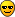# Storing TMatrixD in a root file

Hi!

Iam trying to store TMatrixD objects efficiently in a ROOT file. I first of all tried to just add them to the root file. But because for a TMatrixD object one can not set a “name” this seems not to work. Then I tried a TMap together with a TObjString, but also without success. Finally I tried to use a TTree, where every matrix is stored as a TBranch. But here the filling of the matrices seemed not to work. An additional problem is that i try to do it with Python. Can anybody tell me what the best solution is? So far it looks like this:

``````# prepare ROOT and open the file
from ROOT import TFile, TTree, TString, TObjString, TMatrixD, TPair
from array import array
theFile = TFile(rootfile, "recreate")

theMatrix = TMatrixD(x,y)

# fill the matrices
for matrix in parser.MyMatrices:
for row in range(0,matrix.nrows):
for column in range(0,matrix.ncolumns):
theMatrix[row][column] = float(matrix.data[row][column])
theMatrix.Write()
theFile.Write()

# write and close
theFile.Close()``````

Here the version with tree and branches:

``````# prepare ROOT and open the file
from ROOT import TFile, TTree, TString, TObjString, TMatrixD
from array import array
theFile = TFile(rootfile, "recreate")

#make tree name ROOT save
treename = filename.replace("-","_").split(".")
theTree = TTree(treename, "DataTree")

# create all needed branches
branchBuffers = []
branchname_base = "Matrix"
theMatrix = TMatrixD(x,y)

for timestep in range(0, len(parser.MyMatrices)):

branchBuffers.append(theMatrix)
branchname = branchname_base+"_%s" %timestep
print branchname
tmp = theTree.Branch(branchname, "TMatrixD", branchBuffers[timestep])

# fill the matrices
for branchBuffer, matrix in zip(branchBuffers, parser.MyMatrices):
for row in range(0, matrix.nrows):
for column in range(0, matrix.ncolumns):
theMatrix[row][column] = float(matrix.data[row][column])
print theMatrix[row][column]
branchBuffer = theMatrix[row][column]
theTree.Fill()
theFile.Write()

# write and close
theFile.Close()``````

The  looks strange but worked in the one dimensional case for vectors…
The interesting is, the two branches are in the root file, but when I load both branches the content (the correct values of the matrix) is the same, the right values only appear when I switch between GetEntry(0) and GetEntry(1)…But there only should be one entry in each branch… ?

Cheers,
Stefan

For the non-tree case, you simply use:

For the tree case, you need to make to call theTree.Fill() only once (aka move it one indentation level down)

Cheers,
Philippe

Hi!

Thanks a lot. Yes, this was one major problem. I have still to get used to the python styleOne more thing had to be changed in the case of trees:

``````#make tree name ROOT save
treename = filename.replace("-","_").split(".")
theTree = TTree(treename, "DataTree")

# create all needed branches and buffers
branchBuffers = []
branchname_base = "Matrix"

for timestep in range(0, len(parser.MyMatrices)):

branchBuffers.append(TMatrixD(parser.MyMatrices.nrows, parser.MyMatrices.ncolumns))
branchname = branchname_base+"_%s" %timestep
print branchname
tmp = theTree.Branch(branchname, "TMatrixD", branchBuffers[timestep])

# fill the matrices
for index, matrix in zip(xrange(10000), parser.MyMatrices):
print "--------------------------"
for row in range(0, matrix.nrows):
print "row: "
print row
for column in range(0, matrix.ncolumns):
print float(matrix.data[row][column])
branchBuffers[index][row][column] = float(matrix.data[row][column])
theTree.Fill()
theFile.Write()``````

The “theMatrix” was removed and the content filled directly. Now it works. Just as information for people who might also need a similar solution…

Thanks a lot for the quick answers!
ROOT rulez…